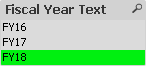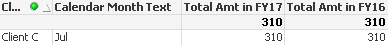# QlikView App Development

Discussion Board for collaboration related to QlikView App Development.

Announcements
Leverage your QlikView investment to modernize BI – see how! Join Group
cancel
Showing results for
Did you mean:
HighlightedContributor

## null value in selected year vs its previous year

Hi there,

I have a question about month over month comparison. What I want to do is to compare the total sales amount between selected fiscal year and the previous year of the selected fiscal year, and then further to calculate growth %.

However, I found that if there is null value in some of the months in selected fiscal year, the total sales amount of this months in its previous fiscal year will be ignored in the calculation. For the example in attached qvw, if Client C has no sales in April and June FY17, the sales amt of April and June in FY16 will be ignored. Please see the set analysis below.

Total Amt in FY16 =

SUM({<[Fiscal Year Text]=,[Fiscal Year] = {\$(=MAX([Fiscal Year])-1)},[Fiscal Month] = p([Fiscal Month]) >} [Amt])

** April and June sales amt are missed in this set analysis.

If I add {1} to possible function, it will include all months. But for the current fiscal year FY18 (fiscal year start at 1 July), I want to show July data only in the table. It seems the {1} not work for current fiscal year.

SUM({<[Fiscal Year Text]=,[Fiscal Year] = {\$(=MAX([Fiscal Year])-1)},[Fiscal Month] = p({1} [Fiscal Month]) >} [Amt])

So, may I ask how can I get the exact total amt for selected year and its previous year?

Expected result:

If select FY18:

total sales amt in FY18: 310

total sales amt in FY17: 310

If select FY17:

total sales amt in FY17: 3050

total sales amt in FY16: 3660 (include April and June data)

Thank you.

6 Replies
HighlightedMaster III

## Re: null value in selected year vs its previous year

Hi Nicole,

try this

SUM({<[Fiscal Year Text]=,[Fiscal Year] = {\$(=MAX([Fiscal Year])-1)},
[Fiscal Month]={'>=\$(=Min([Fiscal Month]))<=\$(=Max([Fiscal Month]))'}>} [Amt]
)Regards,

Antonio

HighlightedPartner

## Re: null value in selected year vs its previous year

hi

use this expression for FY16

SUM({<[Fiscal Year Text]=,[Fiscal Year] = {\$(=MAX([Fiscal Year])-1)},[Fiscal Month] = p({<[Fiscal Year Text]=,[Fiscal Year] = {'\$(=MAX([Fiscal Year])-1)'}>}[Fiscal Month]) >} [Amt])

HighlightedContributor

## Re: null value in selected year vs its previous year

Hi Antonio, Liron,

Thanks for your solutions. But i'm afraid I got a mistake when creating this testing QVW (I put an incorrect order to Fiscal Month). So, the issue is still here when I use the set analysis in the revised QVW.

HighlightedMVP

## Re: null value in selected year vs its previous year

May be try this

SUM({<[Fiscal Year Text]=,[Fiscal Year] = {\$(=MAX([Fiscal Year])-1)},[Fiscal Month] = {"\$(='<=' & Max([Fiscal Month]))"}>} [Amt])

When FY17 is selectedWhen FY18 is selectedHighlightedContributor

## Re: null value in selected year vs its previous year

Hi Sunny,

Thanks for your sharing. It seems June data is missing. I think this is because max fiscal month in FY17 is May, so it return May as max fiscal month in set analysis.Regards,

Nicole

HighlightedMVP

## Re: null value in selected year vs its previous year

May be create a master calendar to handle this issue